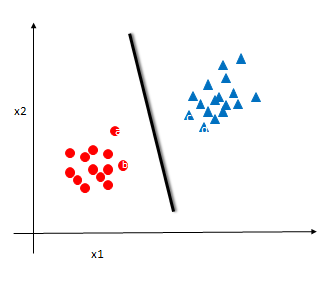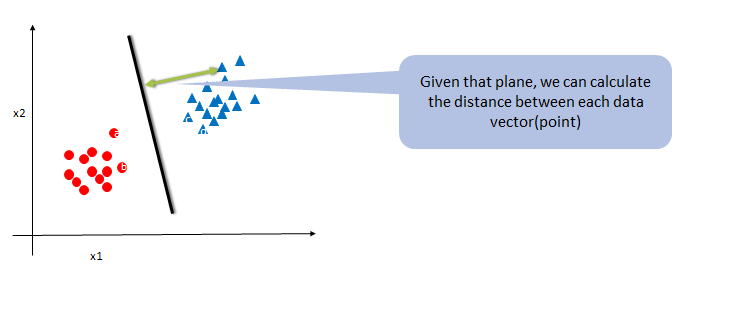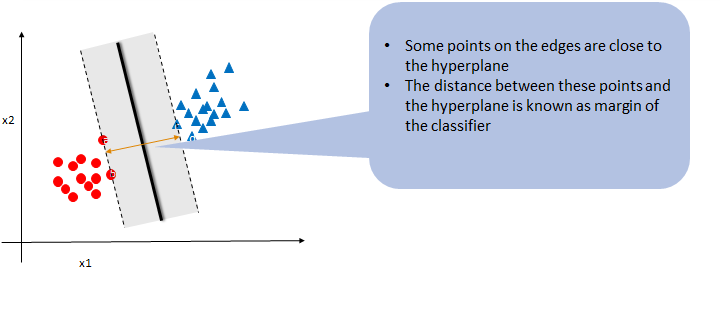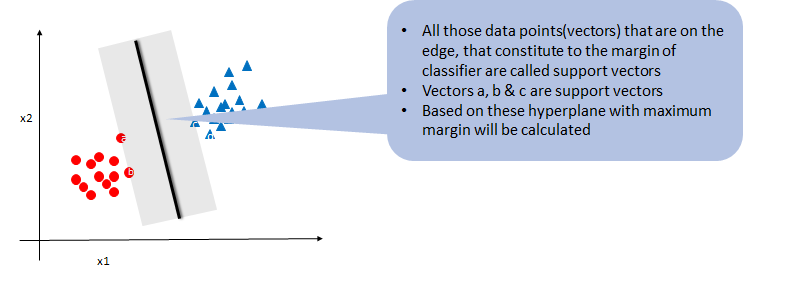• No products in the cart.

# 203.6.3 SVM : The Algorithm

## SVM- The large margin classifier

In previous section, we studied about Simple Classifier

• SVM is all about finding the maximum-margin Classifier.
• Classifier is a generic name, its actually called the hyper plane
• Hyper plane: In 3-dimensional system hyperplanes are the 2-dimensional planes, in 2-dimensional space its hyperplanes are the 1-dimensional lines.
• SVM algorithm makes use of the nearest training examples to derive the classifier with maximum margin
• Each data point is considered as a p-dimensional vector (a list of p numbers)
• SVM uses vector algebra and mathematical optimization to find the optimal hyperplane that has maximum margin

### The SVM Algorithm• If a dataset is linearly separable then we can always find a hyperplane f(x) such that
• For all negative labeled records f(x)<0
• For all positive labeled records f(x)>0
• This hyper plane f(x) is nothing but the linear classifier
• $$f(x)=w_1 x_1+ w_2 x_2 +b$$
• $$f(x)=w^T x+b$$## Math behind SVM Algorithm

### SVM Algorithm – The Math

If you already understood the SVM technique and If you find this slide is too technical, you may want to skip it. The tool will take care of this optimization

1. $$f(x)=w^T x+b$$
2. $$w^T x^+ +b=1$$ and $$w^T x^- +b = -1$$
3. $$x^+ = x^- + \lambda w$$
4. $$w^T x^+ +b=1$$
• $$w^T(x^- + \lambda w)+b=1$$
• $$w^T x^- +\lambda w.w+b=1$$
• $$-1+\lambda w.w=1$$
• $$\lambda = 2/w.w$$
5. $$m =|x^+ – x^-|$$
• $$m=|\lambda w|$$
• $$m=(2/w.w)*|w|$$
• $$m=2/||w||$$
6. Objective is to maximize $$2/||w||$$
• i.e minimize $$||w||$$
7. A good decision boundary should be
• $$w^T x^+ +b>=1$$ for all y=1
• $$w^T x^- +b<=-1$$ for all y=-1
• i.e $$y*(w^T x+b)>=1$$ for all points
8. Now we have the optimization problem with objective and constraints
• minimize $$||w||$$ or $$(½)*||w||^2$$
• With constant $$y(w^T x+b)>=1$$
9. We can solve the above optimization problem to obtain w & b

### SVM Result

• SVM doesn’t output probability. It directly gives which class the new data point belongs to
• For a new point $$x_k$$ calculate \$w^T x_k +b. If this value is positive then the prediction is +1 else -1

### SVM on R

• There are multiple SVM packages available in R. The package “e1071” is the most widely used
• There is a function called svm() within e1071 package
• There are various options within svm() function to customize the training process
library(e1071)
svm_model <- svm(Fraud_id~Total_Amount+Tr_Count_week, data=Transactions_sample)

summary(svm_model)
##
## Call:
## svm(formula = Fraud_id ~ Total_Amount + Tr_Count_week, data = Transactions_sample)
##
##
## Parameters:
##    SVM-Type:  eps-regression
##        cost:  1
##       gamma:  0.5
##     epsilon:  0.1
##
##
## Number of Support Vectors:  24

21st June 2017

### 0 responses on "203.6.3 SVM : The Algorithm"

Statinfer Software Solutions LLP

Software Technology Parks of India,
NH16, Krishna Nagar, Benz Circle,